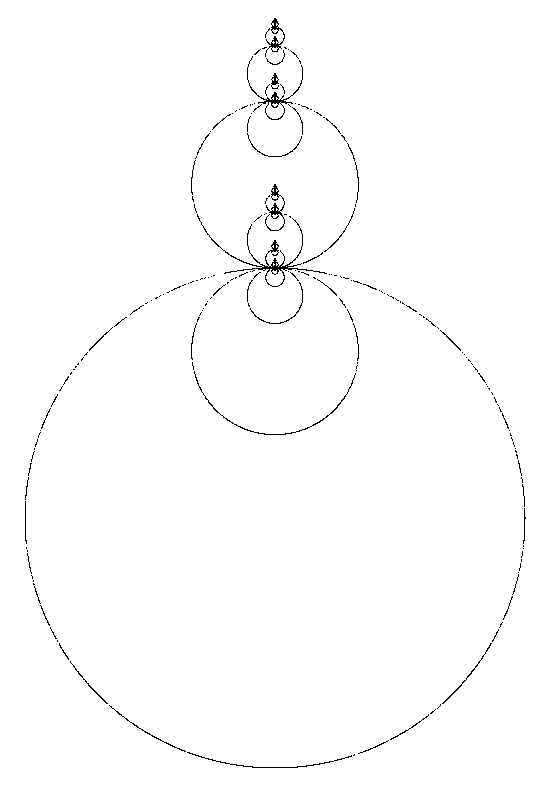# Construction of IFS Fractals of Specific Similarity Dimension

By Roger Bagula
Compiled and graphics by Paul Bourke
26 July 1998For some fractal sets we agree on their dimension: the Cantor set , the von Koch 3 set and the Sierpinski gasket. We also agree that close plane curves have dimension of one. What if we combine one set with another? The union of the two sets will behave as if it had a dimension of the combination set.

On page 160 of Edgar's fractal geometry he defines a Cantor dust as:

1) z = z / 3
2) z = z / 3 + 2/3

Such a set has similarity dimension by Moran's formula of:

3) sc = log(2) / log(3)

It is a Fatou type of set in being unconnected and of dimension less than one. In my work with circle measures I discovered a way to get closed curves:

4) r = m(x,y)

for some measure function m(x,y) and:

5) z = z / r + c(i)

Such closed connected curves have dimension of one exactly. Combination of the Cantor dust and this measure gives an IFS set union with dimension:

6) s = 1 + sc = Hd(curve) + Hd(Cantor dust)

and equations:

7) z = z / m(x,y) + c1 ; p = 1/2
8) z = z / 3 ; p = 1/4
9) z = z / 3 + 2/3 ; p = 1/4

For a circle I use:

10) m(x,y) = sqr(x^2 + y^2)

and c1=-1. I actually have to add a random parity from the set {1,-1} to get both sides of the circle to plot, but the resulting IFS works well. Now, topologically speaking all closed smooth plane curves are dimensionally alike. I had figured out how to make measures that give a lot of different such curves! The regular polygons as inner and outer measures in Hausdorff Space were my best success. The triangle measure is:

11) w = angle(x,y)
12) m(x,y) = Max(Max(-cos(w + 2 * pi / 3), -cos(w + 4 * pi / 3)), -cos(w + 6 * Pi / 3))

This measure gives equilateral triangles instead of circles. Several other measures also work well in these equations. I got a Cantor-Sierpinski by arrangement of the constants. All of these sets share the same dimensionality in theory:

13) set =(Cantor dust set) Union ( curve point set)
14) Hd(set) = Hd(curve) + Hd(Cantor dust)

It works so well I don't have the heart to be too critical, but after seeing the actual sets and changing their shapes at will, I have to say that I doubt that 14) is generally true (specially when I use an hyperbolic measure, for instance). This morning I experimented with the middle fifth Cantor dust set:

15) z = z / 5
16) z = z / 5 + 2/5
17) z = z / 5 + 4/5

And got it to work with a number of curves as well, but used equal probability for the four transforms. The result is not a connected set in the triangular case, but still is in the circle case, even if the circles take a long time to form. I also experimented with replacement of transforms in the Sierpinski sets with alternate triangles to get some pretty fractals. That raised the question of what the dimensionality of connected sets with more than one curve might be: regular transform dimension comes from the Moran equation, but the curves are puzzling:

18) 1 = ((n - int(n / 2)) / 2)^s + (int(n / 2) / m(x,y))^s

Since I have been using :

19) m(x,y) <= 1

We can get an estimate by using the maximum as one and even n:

20) 1 = (n / 2)^s * (1 / 2^s + 1)

If s maxes at two an upper limit is:

21) s = log(5) / (log(2) - log(n / 2))

Which isn't a very good formula for n = 4! Set theory seems to work well until you get to these mixed curves and self-similar sets. I have yet to do some experimentation with this concept, but these Designer fractals a definite step toward making fractals in any desired shape at demand without using a Barnsley type of reduction.

~